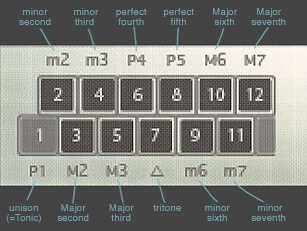## 24,576 Scales feasibleWith AutoTonic it has become possible. now, for the first time in history, to truly modally map ANY existing scale in a logical way (there exist 24,576 scales based on 12 pitches in Western Tonal Music).

It's AutoTonic's 'Scale Modifier' where you can freely assign any desired pattern of intervals per octave …It is well known that 7-note based scales are called "Heptatonic" and 5-note based scales "Pentatonic", but AutoTonic's revolutionary MODAL transposing method looks even beyond that! According to the "Attic Greek" dialect we should be able to classify these other remaining (and mostly unexplored) scales somehow like this:

01 mono- [μονο], Monotonic Scale (Unisons): http://bit.ly/2qKwMID
02 di- [δι], Ditonic scale: http://bit.ly/2q5VG40
03 tri- [τρι], Tritonic scale: http://bit.ly/2rEIB2U
04 tetra- [τετρα], Tetratonic scale: http://bit.ly/2pYE12a
05 penta- [πεντα], Pentatonic Scale: http://bit.ly/1seHFSl
06 hexa- [ἑξα], Hexatonic Scale: http://bit.ly/2q8OH9V
07 hepta- [ἑπτα], Heptatonic Scale: http://bit.ly/2rESttI
08 octa- [ὀκτα], Octattonic Scale: http://bit.ly/2rEsr9J
09 ennea- [ἐννεα], Enneatonic Scale: -Wikipedia n/a-
10 deka- [δεκα], Decatonic Scale: -Wikipedia n/a-
11 hendeka- [ἑνδεκα], Hendecatonic scale: -Wikipedia n/a-
12 dōdeka- [δωδεκα], Dodekatonic scale (Chromatic): -Wikipedia n/a-

Interesting side effect: the more OR less intervals a scale has, the less "permute combination versions" it allows of!Example: While the Hendecatonic scale allows only of 11 combinations (11 "n" places where the 1 missing "r" interval can be) the Hexatonic scale (all combinations of 5 intervals "r" to be placed at 11 positions "n") offers up to 462 combinations, the same for the Heptatonic scale (also 5 intervals "r" at 11 places "n").
That results in…

Monotonic scale: 0 combinations
Ditonic scale: 11 combinations
Tritonic scale: 55 combinations
Tetratonic scale: 165 combinations
Pentatonic scale: 330 combinations
Hexatonic scale: 462 combinations
Heptatonic scale: 462 combinations
Octatonic scale: 330 combinations
Enneatonic scale: 165 combinations
Decatonic scale: 55 combinations
Hendecatonic scale: 11 combinations
Dodekatonic scale: 0 combinations

… and each combination can be mixed with one of the twelve unique Tonics, of course:

In Short:
(1 + 11 + 55 + 165 + 330 + 462 + 462 + 330 + 165 + 55 + 11 + 1) × 12 =
THAT MAKES AN INCREDIBLE TOTAL OF 24,576 SCALES TO CHOOSE FROM.

… in this above linked video you can – for the first time ever – hear every such existing and feasible scale in western tonal music [that's 2,048 SCALES], based on the tonic "C" in 2-Octave-runs (up/down) using a basic Piano-Sound. There exist even more possible combinations than the in the video shown, if also considering all the other TONICs: "C#,D,D#,E,F,F#,G,G#,A,A#,B" [this results in 24,576 feasible SCALES then because the math is: 2^11x12=24,576] but to keep it 'simple' this video shows only the cases with TONIC=C.

## 2,048 Scales x 12 Tonics

Below (link) you can find the complete list of all unique 2,048 combinations of feasible scales based on TONIC=C in AutoTonic – yes, all these scales could also have one of the other tonics C#,D,D#,E,F,F#,G,G#,A,A#,B as well. And since this had already become quite a lengthy video [Total Time=1h:17m:53s] in below's video you may also find the corresponding video markers, which will bring you straight to the corresponding scale types(s): AutoTonic Scale Permutations.html